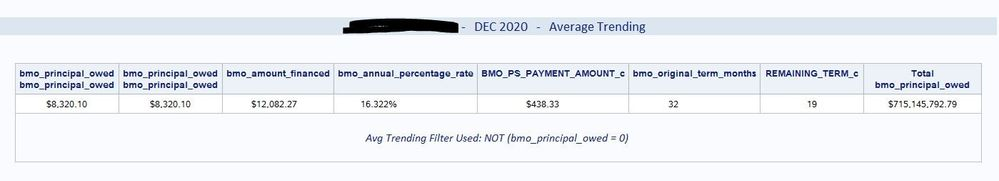## PROC REPORT - header values are being printed twice

Hello,

I am experiencing a weird situation where PROC REPORT is listing the header value twice, i.e. two variable names stacked on top of each other.

Background: I am using a driver/control file that stores information (raw data variable names) for all of our clients, and all of the reporting outputs reference this driver/control file.  When two columns in the PROC REPORT output have the same value, then it lists the variable name twice on top of each other (see attached screenshot).Here is my PROC REPORT code for reference:

```PROC REPORT DATA=&client_number..&client_number._&medte._analysis STYLE(header)=[fontfamily="Albany AMT"] STYLE(column)=[fontfamily="Albany AMT"] ;
SYSECHO "%sysfunc(PROPCASE(%sysfunc(TRANSLATE(&client_name., ' ', '_')))) - Printing Average Trending Statistics" ;
%if %sysevalf(%superq(trending_filter)=, boolean) NE 1 %then %do ; /* test condition to check if where= parameter is blank (1) or has a value (0) */
WHERE (&trending_filter.) ;
%if %upcase(&client_number.) NE GATE %then %do ;
COLUMNS &_gross_balance. &_net_balance. &_amount_financed. &_apr. &_monthly_payment_amount. &_monthly_term. REMAINING_TERM_c &_gross_balance.=total_gross ;
DEFINE &_gross_balance. / MEAN "&_gross_balance." STYLE(column)=[cellwidth=1.4in just=center] ;
DEFINE &_net_balance. / MEAN "&_net_balance." STYLE(column)=[cellwidth=1.4in just=center] ;
%end ;
%else %if %upcase(&client_number.) EQ GATE %then %do ;
COLUMNS &_gross_balance. payoff &_amount_financed. &_apr. &_monthly_payment_amount. &_monthly_term. REMAINING_TERM_c &_gross_balance.=total_gross ;
DEFINE &_gross_balance. / MEAN "&_gross_balance." STYLE(column)=[cellwidth=1.4in just=center] ;
DEFINE payoff / MEAN "payoff" STYLE(column)=[cellwidth=1.4in just=center] ;
%end ;
DEFINE &_amount_financed. / MEAN "&_amount_financed." STYLE(column)=[cellwidth=1.4in just=center] ;
DEFINE &_apr. / MEAN "&_apr." STYLE(column)=[cellwidth=1.2in just=center] ;
DEFINE &_monthly_payment_amount. / MEAN "&_monthly_payment_amount." STYLE(column)=[cellwidth=1.8in just=center] ;
DEFINE &_monthly_term. / MEAN "&_monthly_term." STYLE(column)=[cellwidth=1.2in just=center] FORMAT=3. ;
DEFINE REMAINING_TERM_c / MEAN STYLE(column)=[cellwidth=1.4in just=center] FORMAT=3. ;
DEFINE total_gross / SUM "Total &_gross_balance." STYLE(column)=[cellwidth=1.6in just=center] FORMAT=dollar18.2 ;

COMPUTE AFTER / STYLE=[fontsize=3 fontfamily="Calibri" font_style=italic just=c] ;
LINE " " ;
LINE "Avg Trending Filter Used: &trending_filter." ;
LINE " " ;
ENDCOMP
%end ;
%else %do ;
COLUMNS &_gross_balance. &_net_balance. &_amount_financed. &_apr. &_monthly_payment_amount. &_monthly_term. REMAINING_TERM_c &_gross_balance.=total_gross ;
DEFINE &_gross_balance. / MEAN "&_gross_balance." STYLE(column)=[cellwidth=1.4in just=center] ;
DEFINE &_net_balance. / MEAN "&_net_balance." STYLE(column)=[cellwidth=1.4in just=center] ;
DEFINE &_amount_financed. / MEAN "&_amount_financed." STYLE(column)=[cellwidth=1.4in just=center] ;
DEFINE &_apr. / MEAN "&_apr." STYLE(column)=[cellwidth=1.2in just=center] ;
DEFINE &_monthly_payment_amount. / MEAN "&_monthly_payment_amount." STYLE(column)=[cellwidth=1.8in just=center] ;
DEFINE &_monthly_term. / MEAN "&_monthly_term." STYLE(column)=[cellwidth=1.2in just=center] FORMAT=3. ;
DEFINE REMAINING_TERM_c / MEAN STYLE(column)=[cellwidth=1.4in just=center] FORMAT=3. ;
DEFINE total_gross / SUM "Total &_gross_balance." STYLE(column)=[cellwidth=1.6in just=center] FORMAT=dollar18.2 ;

COMPUTE AFTER / STYLE=[fontsize=3 fontfamily="Calibri" font_style=italic just=c] ;
LINE " " ;
LINE "Avg Trending Filter Used: None" ;
LINE " " ;
ENDCOMP ;
%end ;
RUN ;```

So the issue is that if the values for &_gross_balance. and &_net_balance. are the same, then those variable names are being double printed.

Any help is appreciated, though this is more annoying than anything else.  Thank you!

1 ACCEPTED SOLUTION

Accepted Solutions

## Re: PROC REPORT - header values are being printed twice

Sounds like somewhere you need a requirement for &_gross_balance. and &_net_balance to be different.

That is just another %if &_gross_balance. ne &_net_balance %then %do;

%end;

%else %do; /* you don't include both*/

%end;

blocks where you build Columns statements and Define blocks .

.

3 REPLIES 3

## Re: PROC REPORT - header values are being printed twice

Sounds like somewhere you need a requirement for &_gross_balance. and &_net_balance to be different.

That is just another %if &_gross_balance. ne &_net_balance %then %do;

%end;

%else %do; /* you don't include both*/

%end;

blocks where you build Columns statements and Define blocks .

.

## Re: PROC REPORT - header values are being printed twice

Thank you, @ballardw !

I was hoping to not have to add additional logic to get around this, but it is what it is.  This is what I did and it looks to be working as expected:

```PROC REPORT DATA=&client_number..&client_number._&medte._analysis STYLE(header)=[fontfamily="Albany AMT"] STYLE(column)=[fontfamily="Albany AMT"] ;
SYSECHO "%sysfunc(PROPCASE(%sysfunc(TRANSLATE(&client_name., ' ', '_')))) - Printing Average Trending Statistics" ;
%if %sysevalf(%superq(trending_filter)=, boolean) NE 1 %then %do ; /* test condition to check if where= parameter is blank (1) or has a value (0) */
WHERE (&trending_filter.) ;
%if %upcase(&client_number.) NE GATE %then %do ;
%if %upcase(&_gross_balance.) NE %upcase(&_net_balance.) %then %do ;
COLUMNS &_gross_balance. &_net_balance. &_amount_financed. &_apr. &_monthly_payment_amount. &_monthly_term. REMAINING_TERM_c &_gross_balance.=total_gross ;

DEFINE &_gross_balance. / MEAN "&_gross_balance." STYLE(column)=[cellwidth=1.4in just=center] ;
DEFINE &_net_balance. / MEAN "&_net_balance." STYLE(column)=[cellwidth=1.4in just=center] ;
%end ;
%else %do ;
COLUMNS &_gross_balance. &_gross_balance.=NET_BAL &_amount_financed. &_apr. &_monthly_payment_amount. &_monthly_term. REMAINING_TERM_c &_gross_balance.=total_gross ;

DEFINE &_gross_balance. / MEAN "&_gross_balance." STYLE(column)=[cellwidth=1.4in just=center] ;
DEFINE NET_BAL / MEAN "&_gross_balance." STYLE(column)=[cellwidth=1.4in just=center] ;
%end ;
%end ;
%else %if %upcase(&client_number.) EQ GATE %then %do ;
COLUMNS &_gross_balance. payoff &_amount_financed. &_apr. &_monthly_payment_amount. &_monthly_term. REMAINING_TERM_c &_gross_balance.=total_gross ;

DEFINE &_gross_balance. / MEAN "&_gross_balance." STYLE(column)=[cellwidth=1.4in just=center] ;
DEFINE payoff / MEAN "payoff" STYLE(column)=[cellwidth=1.4in just=center] ;
%end ;```

## Re: PROC REPORT - header values are being printed twice

Glad you could work that out with just a hint.

Discussion stats
• 3 replies
• 356 views
• 2 likes
• 2 in conversation